Neanderthal Pudding Mix

# npm

## d3-scale-chromatic3.0.0 • Public • Published

# d3-scale-chromatic

This module provides sequential, diverging and categorical color schemes designed to work with d3-scale’s d3.scaleOrdinal and d3.scaleSequential. Most of these schemes are derived from Cynthia A. Brewer’s ColorBrewer. Since ColorBrewer publishes only discrete color schemes, the sequential and diverging scales are interpolated using uniform B-splines.

For example, to create a categorical color scale using the Accent color scheme:

var accent = d3.scaleOrdinal(d3.schemeAccent);

To create a sequential discrete nine-color scale using the Blues color scheme:

var blues = d3.scaleOrdinal(d3.schemeBlues);

To create a diverging, continuous color scale using the PiYG color scheme:

var piyg = d3.scaleSequential(d3.interpolatePiYG);

## Installing

If you use npm, npm install d3-scale-chromatic. You can also download the latest release on GitHub. For vanilla HTML in modern browsers, import d3-scale-chromatic from Skypack:

<script type="module">

import {interpolateYlGn} from "https://cdn.skypack.dev/d3-scale-chromatic@3";

const yellow = interpolateYlGn(0); // "rgb(255, 255, 229)"
const yellowGreen = interpolateYlGn(0.5); // "rgb(120, 197, 120)"
const green = interpolateYlGn(1); // "rgb(0, 69, 41)"

</script>

For legacy environments, you can load d3-scale-chromatic’s UMD bundle from an npm-based CDN such as jsDelivr; a d3 global is exported:

<script src="https://cdn.jsdelivr.net/npm/d3-color@3"></script>
<script src="https://cdn.jsdelivr.net/npm/d3-interpolate@3"></script>
<script src="https://cdn.jsdelivr.net/npm/d3-scale-chromatic@3"></script>
<script>

const yellow = d3.interpolateYlGn(0); // "rgb(255, 255, 229)"
const yellowGreen = d3.interpolateYlGn(0.5); // "rgb(120, 197, 120)"
const green = d3.interpolateYlGn(1); // "rgb(0, 69, 41)"

</script>

## API Reference

### Categorical

# d3.schemeCategory10 <>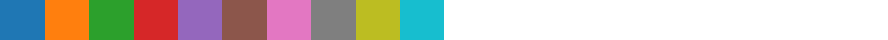An array of ten categorical colors represented as RGB hexadecimal strings.

# d3.schemeAccent <>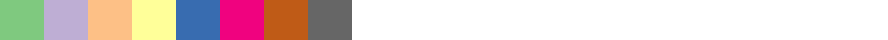An array of eight categorical colors represented as RGB hexadecimal strings.

# d3.schemeDark2 <>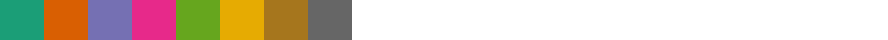An array of eight categorical colors represented as RGB hexadecimal strings.

# d3.schemePaired <>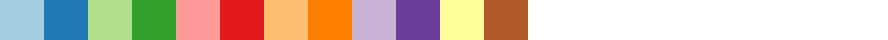An array of twelve categorical colors represented as RGB hexadecimal strings.

# d3.schemePastel1 <>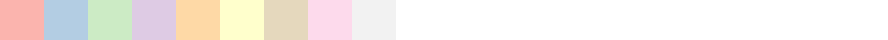An array of nine categorical colors represented as RGB hexadecimal strings.

# d3.schemePastel2 <>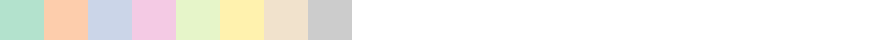An array of eight categorical colors represented as RGB hexadecimal strings.

# d3.schemeSet1 <>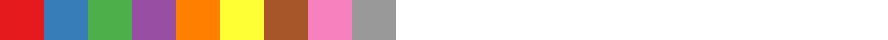An array of nine categorical colors represented as RGB hexadecimal strings.

# d3.schemeSet2 <>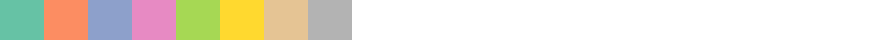An array of eight categorical colors represented as RGB hexadecimal strings.

# d3.schemeSet3 <>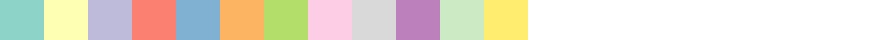An array of twelve categorical colors represented as RGB hexadecimal strings.

# d3.schemeTableau10 <>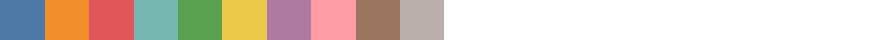An array of ten categorical colors authored by Tableau as part of Tableau 10 represented as RGB hexadecimal strings.

### Diverging

Diverging color schemes are available as continuous interpolators (often used with d3.scaleSequential) and as discrete schemes (often used with d3.scaleOrdinal). Each discrete scheme, such as d3.schemeBrBG, is represented as an array of arrays of hexadecimal color strings. The kth element of this array contains the color scheme of size k; for example, d3.schemeBrBG contains an array of nine strings representing the nine colors of the brown-blue-green diverging color scheme. Diverging color schemes support a size k ranging from 3 to 11.

# d3.interpolateBrBG(t) <>
# d3.schemeBrBG[k]Given a number t in the range [0,1], returns the corresponding color from the “BrBG” diverging color scheme represented as an RGB string.

# d3.interpolatePRGn(t) <>
# d3.schemePRGn[k]Given a number t in the range [0,1], returns the corresponding color from the “PRGn” diverging color scheme represented as an RGB string.

# d3.interpolatePiYG(t) <>
# d3.schemePiYG[k]Given a number t in the range [0,1], returns the corresponding color from the “PiYG” diverging color scheme represented as an RGB string.

# d3.interpolatePuOr(t) <>
# d3.schemePuOr[k]Given a number t in the range [0,1], returns the corresponding color from the “PuOr” diverging color scheme represented as an RGB string.

# d3.interpolateRdBu(t) <>
# d3.schemeRdBu[k]Given a number t in the range [0,1], returns the corresponding color from the “RdBu” diverging color scheme represented as an RGB string.

# d3.interpolateRdGy(t) <>
# d3.schemeRdGy[k]Given a number t in the range [0,1], returns the corresponding color from the “RdGy” diverging color scheme represented as an RGB string.

# d3.interpolateRdYlBu(t) <>
# d3.schemeRdYlBu[k]Given a number t in the range [0,1], returns the corresponding color from the “RdYlBu” diverging color scheme represented as an RGB string.

# d3.interpolateRdYlGn(t) <>
# d3.schemeRdYlGn[k]Given a number t in the range [0,1], returns the corresponding color from the “RdYlGn” diverging color scheme represented as an RGB string.

# d3.interpolateSpectral(t) <>
# d3.schemeSpectral[k]Given a number t in the range [0,1], returns the corresponding color from the “Spectral” diverging color scheme represented as an RGB string.

### Sequential (Single Hue)

Sequential, single-hue color schemes are available as continuous interpolators (often used with d3.scaleSequential) and as discrete schemes (often used with d3.scaleOrdinal). Each discrete scheme, such as d3.schemeBlues, is represented as an array of arrays of hexadecimal color strings. The kth element of this array contains the color scheme of size k; for example, d3.schemeBlues contains an array of nine strings representing the nine colors of the blue sequential color scheme. Sequential, single-hue color schemes support a size k ranging from 3 to 9.

# d3.interpolateBlues(t) <>
# d3.schemeBlues[k]Given a number t in the range [0,1], returns the corresponding color from the “Blues” sequential color scheme represented as an RGB string.

# d3.interpolateGreens(t) <>
# d3.schemeGreens[k]Given a number t in the range [0,1], returns the corresponding color from the “Greens” sequential color scheme represented as an RGB string.

# d3.interpolateGreys(t) <>
# d3.schemeGreys[k]Given a number t in the range [0,1], returns the corresponding color from the “Greys” sequential color scheme represented as an RGB string.

# d3.interpolateOranges(t) <>
# d3.schemeOranges[k]Given a number t in the range [0,1], returns the corresponding color from the “Oranges” sequential color scheme represented as an RGB string.

# d3.interpolatePurples(t) <>
# d3.schemePurples[k]Given a number t in the range [0,1], returns the corresponding color from the “Purples” sequential color scheme represented as an RGB string.

# d3.interpolateReds(t) <>
# d3.schemeReds[k]Given a number t in the range [0,1], returns the corresponding color from the “Reds” sequential color scheme represented as an RGB string.

### Sequential (Multi-Hue)

Sequential, multi-hue color schemes are available as continuous interpolators (often used with d3.scaleSequential) and as discrete schemes (often used with d3.scaleOrdinal). Each discrete scheme, such as d3.schemeBuGn, is represented as an array of arrays of hexadecimal color strings. The kth element of this array contains the color scheme of size k; for example, d3.schemeBuGn contains an array of nine strings representing the nine colors of the blue-green sequential color scheme. Sequential, multi-hue color schemes support a size k ranging from 3 to 9.

# d3.interpolateTurbo(t) <>Given a number t in the range [0,1], returns the corresponding color from the “turbo” color scheme by Anton Mikhailov.

# d3.interpolateViridis(t) <>Given a number t in the range [0,1], returns the corresponding color from the “viridis” perceptually-uniform color scheme designed by van der Walt, Smith and Firing for matplotlib, represented as an RGB string.

# d3.interpolateInferno(t) <>Given a number t in the range [0,1], returns the corresponding color from the “inferno” perceptually-uniform color scheme designed by van der Walt and Smith for matplotlib, represented as an RGB string.

# d3.interpolateMagma(t) <>Given a number t in the range [0,1], returns the corresponding color from the “magma” perceptually-uniform color scheme designed by van der Walt and Smith for matplotlib, represented as an RGB string.

# d3.interpolatePlasma(t) <>Given a number t in the range [0,1], returns the corresponding color from the “plasma” perceptually-uniform color scheme designed by van der Walt and Smith for matplotlib, represented as an RGB string.

# d3.interpolateCividis(t) <>Given a number t in the range [0,1], returns the corresponding color from the “cividis” color vision deficiency-optimized color scheme designed by Nuñez, Anderton, and Renslow, represented as an RGB string.

# d3.interpolateWarm(t) <>Given a number t in the range [0,1], returns the corresponding color from a 180° rotation of Niccoli’s perceptual rainbow, represented as an RGB string.

# d3.interpolateCool(t) <>Given a number t in the range [0,1], returns the corresponding color from Niccoli’s perceptual rainbow, represented as an RGB string.

# d3.interpolateCubehelixDefault(t) <>Given a number t in the range [0,1], returns the corresponding color from Green’s default Cubehelix represented as an RGB string.

# d3.interpolateBuGn(t) <>
# d3.schemeBuGn[k]Given a number t in the range [0,1], returns the corresponding color from the “BuGn” sequential color scheme represented as an RGB string.

# d3.interpolateBuPu(t) <>
# d3.schemeBuPu[k]Given a number t in the range [0,1], returns the corresponding color from the “BuPu” sequential color scheme represented as an RGB string.

# d3.interpolateGnBu(t) <>
# d3.schemeGnBu[k]Given a number t in the range [0,1], returns the corresponding color from the “GnBu” sequential color scheme represented as an RGB string.

# d3.interpolateOrRd(t) <>
# d3.schemeOrRd[k]Given a number t in the range [0,1], returns the corresponding color from the “OrRd” sequential color scheme represented as an RGB string.

# d3.interpolatePuBuGn(t) <>
# d3.schemePuBuGn[k]Given a number t in the range [0,1], returns the corresponding color from the “PuBuGn” sequential color scheme represented as an RGB string.

# d3.interpolatePuBu(t) <>
# d3.schemePuBu[k]Given a number t in the range [0,1], returns the corresponding color from the “PuBu” sequential color scheme represented as an RGB string.

# d3.interpolatePuRd(t) <>
# d3.schemePuRd[k]Given a number t in the range [0,1], returns the corresponding color from the “PuRd” sequential color scheme represented as an RGB string.

# d3.interpolateRdPu(t) <>
# d3.schemeRdPu[k]Given a number t in the range [0,1], returns the corresponding color from the “RdPu” sequential color scheme represented as an RGB string.

# d3.interpolateYlGnBu(t) <>
# d3.schemeYlGnBu[k]Given a number t in the range [0,1], returns the corresponding color from the “YlGnBu” sequential color scheme represented as an RGB string.

# d3.interpolateYlGn(t) <>
# d3.schemeYlGn[k]Given a number t in the range [0,1], returns the corresponding color from the “YlGn” sequential color scheme represented as an RGB string.

# d3.interpolateYlOrBr(t) <>
# d3.schemeYlOrBr[k]Given a number t in the range [0,1], returns the corresponding color from the “YlOrBr” sequential color scheme represented as an RGB string.

# d3.interpolateYlOrRd(t) <>
# d3.schemeYlOrRd[k]Given a number t in the range [0,1], returns the corresponding color from the “YlOrRd” sequential color scheme represented as an RGB string.

### Cyclical

# d3.interpolateRainbow(t) <>Given a number t in the range [0,1], returns the corresponding color from d3.interpolateWarm scale from [0.0, 0.5] followed by the d3.interpolateCool scale from [0.5, 1.0], thus implementing the cyclical less-angry rainbow color scheme.

# d3.interpolateSinebow(t) <>Given a number t in the range [0,1], returns the corresponding color from the “sinebow” color scheme by Jim Bumgardner and Charlie Loyd.

## Keywords

### Install

npm i d3-scale-chromatic

### Repository

github.com/d3/d3-scale-chromatic

### Homepage

d3js.org/d3-scale-chromatic/

1,181,064

3.0.0